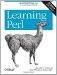# Section B.9. Mathematics

### B.9. Mathematics

Perl can do just about any kind of mathematics you can dream up.

All of the basic mathematical functions (square root, cosine, logarithm, absolute value, and many others) are available as built-in functions. See the perlfunc manpage for details. Some others (like tangent or base-10 logarithm) are omitted, but those can be created from the basic ones or loaded from a module. (See the POSIX module for many common math functions.)

#### B.9.2. Imaginary and Complex Numbers

Though the core of Perl doesn't directly support them, modules are available for working with complex numbers. These overload the normal operators and functions, so you can multiply with * and get a square root with sqrt even when using complex numbers. See the Math::Complex module.

#### B.9.3. Large and High-Precision Numbers

You can do math with arbitrarily large numbers with an arbitrary number of digits of accuracy. For example, you could calculate the factorial of 2,000, or determine p to 10,000 digits. See the Math::BigInt and Math::BigFloat modules.Learning Perl, 5th Edition
ISBN: 0596520107
EAN: 2147483647
Year: 2003
Pages: 232

Similar book on Amazon